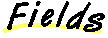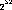`Packages  This Package  Prev  Next  Index  `

# §1.8 Class Integer

```public  final  class  java.lang.Integer
extends  java.lang.Number  (I-§1.11)
{
// Fields
public final static int MAX_VALUE;	§1.8.1
public final static int MIN_VALUE;	§1.8.2

// Constructors
public Integer(int  value);	§1.8.3
public Integer(String  s);	§1.8.4

// Methods
public double doubleValue();	§1.8.5
public boolean equals(Object  obj);	§1.8.6
public float floatValue();	§1.8.7
public static Integer getInteger(String  nm);	§1.8.8
public static Integer getInteger(String  nm, int  val);	§1.8.9
public static Integer getInteger(String  nm, Integer  val);	§1.8.10
public int hashCode();	§1.8.11
public int intValue();	§1.8.12
public long longValue();	§1.8.13
public static int parseInt(String  s);	§1.8.14
public static int parseInt(String  s, int  radix);	§1.8.15
public static String toBinaryString(int  i);	§1.8.16
public static String toHexString(int  i);	§1.8.17
public static String toOctalString(int  i);	§1.8.18
public String toString();	§1.8.19
public static String toString(int  i);	§1.8.20
public static String  toString(int  i, int  radix);	§1.8.21
public static Integer valueOf(String  s);	§1.8.22
public static Integer valueOf(String  s, int  radix);	§1.8.23
}
```
This class wraps a value of the primitive type int in an object. An object of type Integer contains a single field whose type is int.

In addition, this class provides a number of methods for converting an int to a String and a String to an int, as well as other constants and methods useful when dealing with an int.

##### MAX_VALUE

```public final static int MAX_VALUE = 2147483647 ```
The largest value of type int.

### MIN_VALUE

```public final static int MIN_VALUE = -2147483648 ```
The smallest value of type int.

##### Integer

```public Integer(int value) ```
Constructs a newly allocated Integer object that represents the primitive int argument.
Parameters:
```value - ```the value to be reprsented by the Integer

### Integer

```public Integer(String s) ``` ```throws NumberFormatException ```
Constructs a newly allocated Integer object that represents the value represented by the string The string is converted to an int value as if by the valueOf method (I-§1.8.22).

Parameters:
```s - ```the String to be converted to an Integer
Throws
NumberFormatException (I-§1.41)
If the String does not contain a parsable integer.

##### doubleValue

```public double doubleValue() ```
Returns:
The int value represented by this object is converted to type double and the result of the conversion is returned.
Overrides:
doubleValue in class Number (I-§1.11.1).

### equals

```public boolean equals(Object obj) ```
The result is true if and only if the argument is not null and is an Integer object that contains the same int value as this object.
Parameters:
```obj - ```the object to compare with
Returns:
true if the objects are the same; false otherwise.
Overrides:
equals in class Object (I-§1.12.3).

### floatValue

```public float floatValue() ```
Returns:
the int value represented by this object is converted to type float and the result of the conversion is returned..
Overrides:
floatValue in class Number (I-§1.11.2).

### getInteger

```public static Integer getInteger(String nm) ```
Determines the integer value of the system property with the specified name.
The first argument is treated as the name of a system property to be obtained as if by the method System.getProperty (I-§1.18.10). The string value of this property is then interpreted as an integer value and an Integer object representing this value is returned. The full details of the possible numeric formats are given in I-§1.8.10.

If there is no property with the specified name, or if the property does not have the correct numeric format, then null is returned.
Parameters:
```nm - ```property name
Returns:
the Integer value of the property.

### getInteger

```public static Integer getInteger(String nm, int val) ```
Determines the integer value of the system property with the specified name.
The first argument is treated as the name of a system property to be obtained as if by the method System.getProperty (I-§1.18.10). The string value of this property is then interpreted as an integer value and an Integer object representing this value is returned. The full details of the possible numeric formats are given in I-§1.8.10.

If there is no property with the specified name, or if the property does not have the correct numeric format, then an Integer object that represents the value of the second argument is returned.
Parameters:
```nm - ```property name
```val - ```default value
Returns:
the Integer value of the property.

### getInteger

```public static Integer getInteger(String nm, Integer val) ```
Determines the integer value of the system property with the specified name.
The first argument is treated as the name of a system property to be obtained as if by the method System.getProperty (I-§1.18.10). The string value of this property is then interpreted as an integer value and an Integer object representing this value is returned.

If the property value begins with "0x" or "#", not followed by a minus sign, the rest of it is parsed as a hexadecimal integer exactly as for the method Integer.valueOf (I-§1.8.23) with radix 16.

If the property value begins with "0" then it is parsed as an octal integer exactly as for the method Integer.valueOf (I-§1.8.23) with radix 8.

Otherwise the property value is parsed as a decimal integer exactly as for the method Integer.valueOf (I-§1.8.23) with radix 10.

The second argument is the default value. If there is no property of the specified name, or if the property does not have the correct numeric format, then the second argument is returned.
Parameters:
```nm - ```property name
```val - ```default value
Returns:
the Integer value of the property.

### hashCode

```public int hashCode() ```
Returns:
a hash code value for this object.
Overrides:
hashCode in class Object (I-§1.12.6).

### intValue

```public int intValue() ```
Returns:
The int value represented by this object is returned.
Overrides:
intValue in class Number (I-§1.11.3).

### longValue

```public long longValue() ```
Returns:
The int value represented by this object is converted to type long and the result of the conversion is returned..
Overrides:
longValue in class Number (I-§1.11.4).

### parseInt

```public static int parseInt(String s) ``` ```throws NumberFormatException ```
Parses the string argument as a signed decimal integer. The characters in the string must all be decimal digits, except that the first character may be an ASCII minus sign '-' to indicate a negative value.
Parameters:
```s - ```a string
Returns:
the integer represented by the argument in decimal.
Throws
NumberFormatException (I-§1.41)
If the string does not contain a parsable integer.

### parseInt

```public static int parseInt(String s, int radix) ``` ```throws NumberFormatException ```
Parses the string argument as a signed integer in the radix specified by the second argument. The characters in the string must all be digits of the specified radix (as determined by whether Character.digit (I-§1.2.7) returns a nonnegative value), except that the first character may be the an ASCII minus sign '-' to indicate a negative value. The resulting integer value is returned.

Parameters:
```s - ```the String containing the integer
```radix - ```the radix to be used
Returns:
the integer represented by the string argument in the specified radix.
Throws
NumberFormatException (I-§1.41)
If the string does not contain a parsable integer.

### toBinaryString1

```public static String toBinaryString(int i) ```
Creates a string representation of the integer argument as an unsigned integer in base 2.
The unsigned integer value is the argument plusif the argument is negative; otherwise it is equal to the argument. This value is converted to a string of ASCII digits in binary (base 2) with no extra leading 0's.
Parameters:
```i - ```an integer
Returns:
the string representation of the unsigned integer value represented by the argument in binary (base 2).

### toHexString2

```public static String toHexString(int i) ```
Creates a string representation of the integer argument as an unsigned integer in base 16.
The unsigned integer value is the argument plusif the argument is negative; otherwise it is equal to the argument. This value is converted to a string of ASCII digits in hexadecimal (base 16) with no extra leading 0's.
Parameters:
```i - ```an integer
Returns:
the string representation of the unsigned integer value represented by the argument in hexadecimal (base 16).

### toOctalString3

```public static String toOctalString(int i) ```
Creates a string representation of the integer argument as an unsigned integer in base 8.
The unsigned integer value is the argument plusif the argument is negative; otherwise it is equal to the argument. This value is converted to a string of ASCII digits in octal (base 8) with no extra leading 0's.
Parameters:
```i - ```an integer
Returns:
the string representation of the unsigned integer value represented by the argument in octal (base 8).

### toString

```public String toString() ```
Returns:
a string representation of the value of this object in base 10.
Overrides:
toString in class Object (I-§1.12.9).

### toString

```public static String toString(int i) ```
Parameters:
```i - ```an integer to be converted
Returns:
a string representation of the argument in base 10.

### toString

```public static String toString(int i, int radix) ```
Creates a string representation of the first argument in the radix specified by the second argument.
If the radix is smaller than Character.MIN_RADIX (I-§1.2.3) or larger than Character.MAX_RADIX (I-§1.2.1), then the radix 10 is used instead.

If the first argument is negative, the first element of the result is the ASCII minus characer '-' . If the first argument is not negative, no sign character appears in the result. The following ASCII characters are used as digits:
0123456789abcdefghijklmnopqrstuvwxyz
Parameters:
```i - ```an integer
```radix - ```the radix
Returns:
a string representation of the argument in the specified radix.

### valueOf

```public static Integer valueOf(String s) ``` ```throws NumberFormatException ```
Parameters:
```s - ```the string to be parsed
Returns:
a newly constructed Integer initialized to the value represented by the string argument.
Throws
NumberFormatException (I-§1.41)
If the string does not contain a parsable integer.

### valueOf

```public static Integer valueOf(String s, int radix) ``` ```throws NumberFormatException ```
Parameters:
```s - ```the string to be parsed
Returns:
a newly constructed Integer initialized to the value represented by the string argument in the specified radix.
Throws
NumberFormatException (I-§1.41)
If the String does not contain a parsable integer.

1 This method is new in Java 1.1

2 This method is new in Java 1.1.

3 This method is new in Java 1.1.

`Packages  This Package  Prev  Next  Index`
Java API Document (HTML generated by dkramer on April 22, 1996)